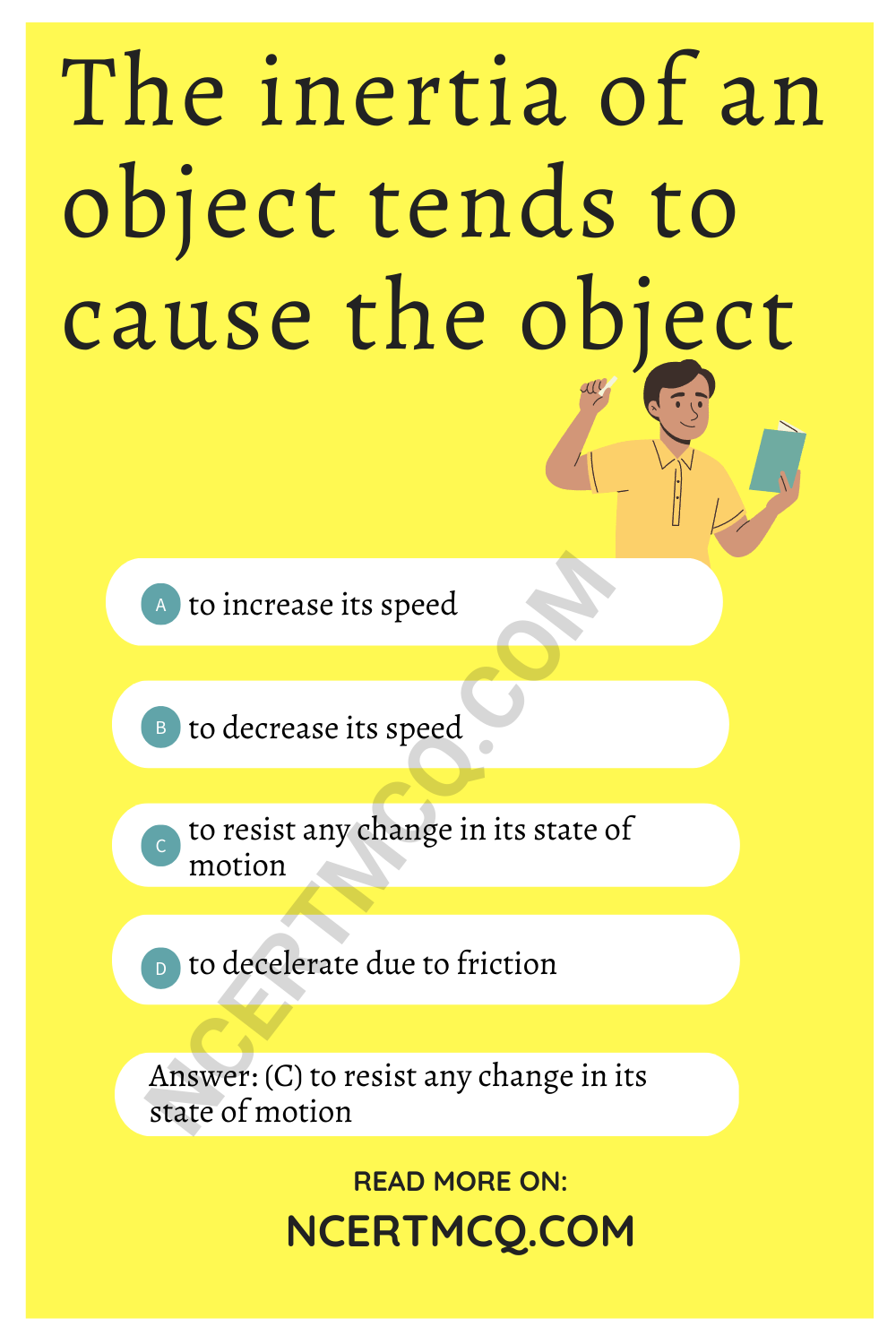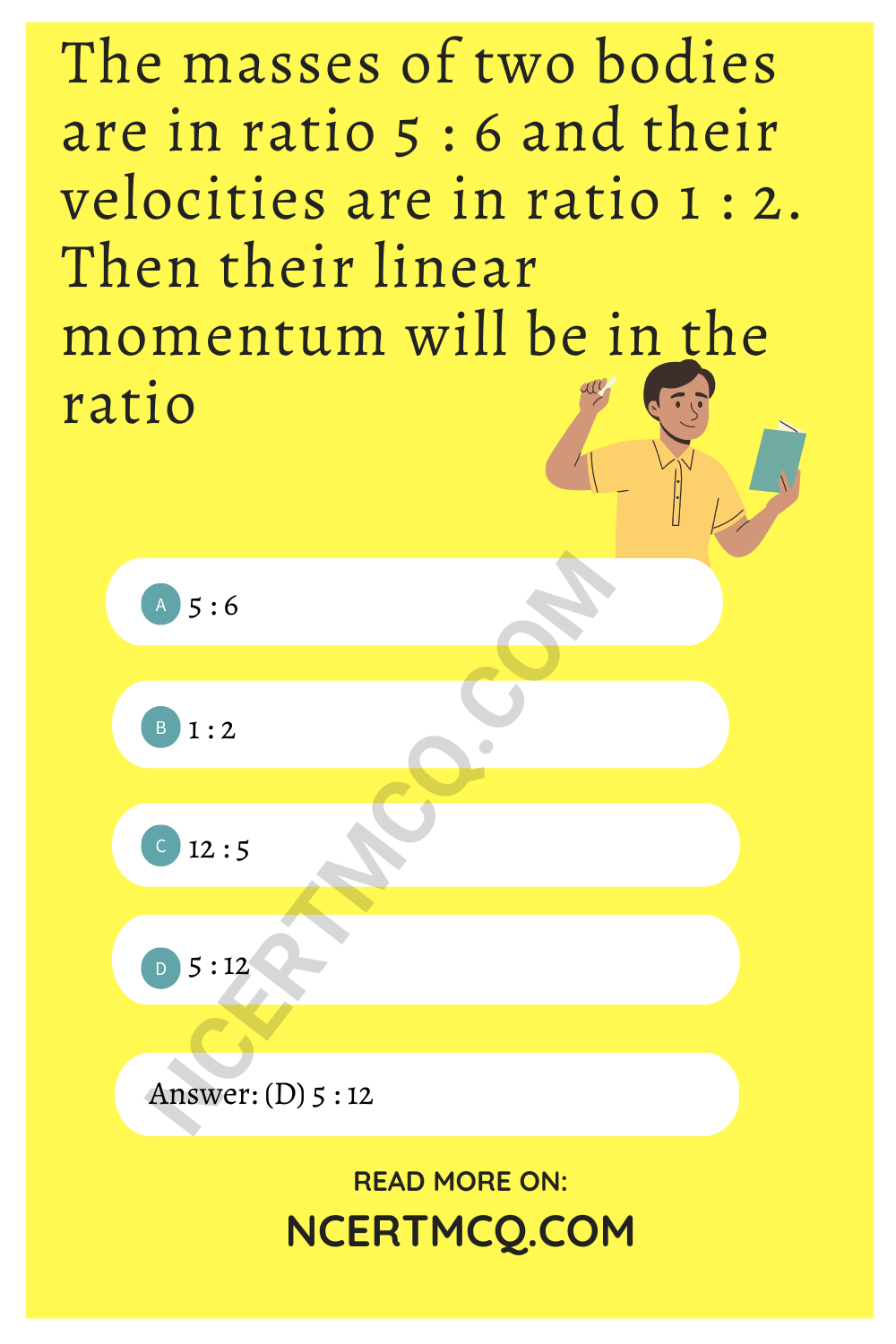Check the below Online Education NCERT MCQ Questions for Class 9 Science Chapter 9 Force and Laws of Motion with Answers Pdf free download. MCQ Questions for Class 9 Science with Answers were prepared based on the latest exam pattern. We have Provided Force and Laws of Motion Class 9 Science MCQs Questions with Answers to help students understand the concept very well. https://ncertmcq.com/mcq-questions-for-class-9-science-with-answers/

You can refer to NCERT Solutions for Class 9 Science Chapter 9 Force and Laws of Motion to revise the concepts in the syllabus effectively and improve your chances of securing high marks in your board exams.

## Force and Laws of Motion Class 9 MCQs Questions with Answers

Force And Laws Of Motion Class 9 MCQ Question 1.
Which of the following statements is not correct for an object moving along a straight path in an accelerated motion?
(a) Its speed keeps changing
(b) Its velocity always changes
(c) It always goes away from the Earth
(d) A force is always acting on it

Answer: (d) A force is always acting on it

Force And Laws Of Motion MCQ Question 2.
According to the third law of motion, action and reaction
(a) always act on the same body
(b) always act on different bodies in opposite directions
(c) have same magnitude and directions
(d) act on either body at normal to each other

Answer: (b) always act on different bodies in opposite directions

Class 9 Science Chapter 9 MCQ Question 3.
A goalkeeper in a game of football pulls his hands backwards after holding the ball shot at the goal. This enables the goalkeeper to
(a) exert larger force on the ball
(b) reduce the force exerted by the balls on the hands
(c) increase the rate of change of momentum
(d) decrease the rate of change of momentum

Answer: (d) decrease the rate of change of momentum

Class 9 Force And Laws Of Motion MCQ Question 4.
The inertia of an object tends to cause the object
(a) to increase its speed
(b) to decrease its speed
(c) to resist any change in its state of motion
(d) to decelerate due to friction

Answer: (c) to resist any change in its state of motionPhysics MCQ Questions Class 9 Force And Laws Of Motion With Answers Question 5.
A passenger in a moving train tosses a coin which falls behind him. It means that motion of the train is
(a) accelerated
(b) uniform
(c) retarded
(d) along circular tracks

Class 9 Science Ch 9 MCQ Question 6.
An object of mass 2 kg is sliding with a constant velocity of 4 ms-1 on a frictionless horizontal table. The force required to keep the object moving with the same velocity is
(a) 32 N
(b) 0 N
(c) 2 N
(d) 8 N

From newton’s Ist law of motion, since there is no external force acting on the object it will remain in its original state of motion. Hence, zero force is required to keep the object moving with constant velocity, correct answer is B.

Ch 9 Science Class 9 MCQ Question 7.
Rocket works on the principle of conservation of
(a) mass
(b) energy
(c) momentum
(d) velocity

MCQ Of Force And Laws Of Motion Question 8.
A water tanker filled up to $$\frac{2}{3}$$ of its height is moving with a uniform speed. On a sudden application of brakes, the water in the tank would
(a) move backward
(b) move forward
(c) be unaffected
(d) rise upwards

Force And Laws Of Motion MCQ Class 9 Question 9.
If the mass of a body is doubled and its velocity becomes half, then the linear momentum of the body will
(a) remain same
(b) become double
(c) become half
(d) become four times.

Class 9 Chapter 9 Science MCQ Question 10.
When a number of forces acting simultaneously on a body bring about a change in its state of rest or of uniform motion in a straight line, then these forces acting on the body are said to be
(a) balanced forces
(b) equal forces
(c) unbalanced forces
(d) opposite forces

MCQ On Force And Laws Of Motion Question 11.
When a car at high speed makes a sharp turn, the driver in a car tends to get thrown to the side opposite to the turn. This is due to the
(a) inertia of motion
(b) inertia of time
(c) inertia of rest
(d) inertia of direction

MCQ On Force And Laws Of Motion Class 9 Question 12.
A man is standing on a boat in still water. If he walks towards the shore, then the boat will
(a) move away from the shore
(b) move towards the shore
(c) remain stationary
(d) none of these

Answer: (a) move away from the shore

Class 9 Ch 9 Science MCQ Question 13.
Which of the following is an incorrect statement?
(a) Mass is measure of inertia of a body.
(b) Newton’s first law of motion is the law of inertia.
(c) Unbalanced force produces constant velocity.
(d) Newton’s third law talks about the direction of the force.

Answer: (c) Unbalanced force produces constant velocity.

Chapter 9 Science Class 9 MCQ Question 14.
A ball is thrown vertically upward in a train moving with uniform velocity. The ball will
(a) fall behind the thrower
(b) fall ahead of the thrower
(c) return back to the thrower
(d) fall on the left of the thrower

Answer: (c) return back to the thrower

Class 9 Physics Chapter 2 MCQ Question 15.
Which of the following is not an application of conservation of linear momentum?
(a) While firing a bullet, the gun must be held tight to the shoulder
(b) When a man jumps from a boat to the shore
(c) A rocket explodes on midway from the ground
(d) A body suspended from the hook of a spring balanced in a lift which is accelerated downward

Answer: (c) A rocket explodes on midway from the ground

Question 16.
When we stop pedalling, the bicycle begins to slow down. This is because of the
(a) Frictional force acting along the direction of motion of bicycle
(b) Air resistance which is in the direction of motion
(c) Frictional force acting opposite to the direction of motion of bicycle by the road
(d) Nature of the bicycle to stop after some time

Answer: (d) Nature of the bicycle to stop after some time

Question 17.
Inertia is the property of a body by virtue of which, it cannot change by itself
(a) its state of rest
(b) its steady state of uniform motion
(c) its direction of motion
(d) all of these.

Question 18.
An athlete does not come to rest immediately after crossing the winning line due to the
(a) inertia of motion
(b) inertia of rest
(c) inertia of direction
(d) none of these

Question 19.
A bullet of mass A and velocity B is fired into a wooden block of mass C. If the bullet gets embedded in the wooden block, then the magnitude of velocity of the system just after the collision will be
(a) $$\frac{A+B}{AC}$$
(b) $$\frac{A+C}{B+C}$$
(c) $$\frac{AC}{B+C}$$
(d) $$\frac{AB}{A+C}$$

Answer: (d) $$\frac{AB}{A+C}$$

Question 20.
The masses of two bodies are in ratio 5 : 6 and their velocities are in ratio 1 : 2. Then their linear momentum will be in the ratio
(a) 5 : 6
(b) 1 : 2
(c) 12 : 5
(d) 5 : 12Fill in the blanks

1. The momentum of an object is product of its mass and ……………..

2. According to second law of motion “the rate of change of momentum is directly proportional to ……………… applied”.

3. According to law of conservation of momentum “the total momentum of an isolated system is always ……………..

4. Recoiling of gun is an example of ………….. law of motion.

5. According to third law of motion “to every action there is an equal and ……………… reaction and they act on two different bodies”.

6. The forces are said to be balanced, if net force is ……………..

7. When a carpet is beaten with a stick, dust particle comes out. This phenomenon is an example of …………….. law of motion.

Match the following columns

 Column A Column B (a) Action and reaction (i) Balanced forces (b) Cricket player lowers his hand while catching the ball (ii) Newton’s first law (c) Rocket propulsion (iii) Mass (d) Resultant force is zero (iv) Newton’s second law (e) A ball thrown upward in a train moving with uniform velocity returns to the thrower. (v) Inertia (f) SI unit of momentum (vi) Newton’s third law (g) Tendency of a body to resist change in its state of motion or rest. (vii) Conservation of linear momentum (h) Inertia of a body depends on it (viii) kg m/s²

 Column A Column B (a) Action and reaction (vi) Newton’s third law (b) Cricket player lowers his hand while catching the ball (iv) Newton’s second law (c) Rocket propulsion (vii) Conservation of linear momentum (d) Resultant force is zero (i) Balanced forces (e) A ball thrown upward in a train moving with uniform velocity returns to the thrower. (ii) Newton’s first law (f) SI unit of momentum (viii) kg m/s² (g) Tendency of a body to resist change in its state of motion or rest. (v) Inertia (h) Inertia of a body depends on it (iii) Mass

Complete the crossword given below:Across:
2. SI unit of force
3. Force which opposes relative motion
5. Measure of inertia
7. First law is also known as law of

Down:
1. Product of mass and velocity
4. Natural tendency of an object to resist a change in its states of motion or rest
6. Magnitude of resultant if forces are balanced